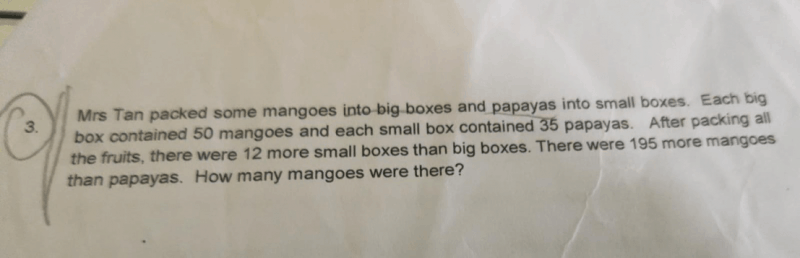# Question1box of mangoes= 50

1box of papayas= 35

Difference 1: 50-35 = 15

12 more small boxes = 12×35 = 420

Difference 2: (this is difference between 12 more small boxes of papayas and 195 more mangoes)

Difference 2: 420 – (-195) = 420+195 = 615

Third: 615 / 15 = 41 sets (or 41 boxes in this case)

Mangoes= 41 boxes x 50 = 2050

Checking step:

Papayas= 41 boxes + 12 boxes more = 53 boxes

53 boxes x 35 = 1855

2050-1855 = 195 (tally with 195 more mangoes stated in question)

0 Replies 0 Likes